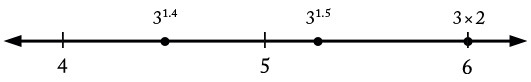# Checking a calculation of a decimal exponent

Alignments to Content Standards: N-RN.A.2

Alicia and Zara are scientists working together. Alicia uses a calculator to evaluate $3^{1.4}$ and gets an answer of $6.473$. Zara thinks for a moment, makes some calculations on paper, and says "That cannot be right, because $3^{1.4}$ must be less than $6$."

Find some hand calculations which show that, as Zara says, $3^{1.4}$ must be less than $6$.

## IM Commentary

This task is primarily suited for instruction as written, though could be used for assessment in settings where varied answers (as in solutions below) can easily be checked, in particular for formative assessment. The purpose of the task is to connect properties of fractional exponents with ordering of real numbers. One can place the numbers on the number line to emphasize this.

Students may be allowed to use their own calculator but not the exponential button (in a classroom setting) or supplied with a rudimentary calculator (in an assessment setting).

Most solutions use the facts that if $\frac{p}{q} < \frac{r}{s}$ then $a^{\frac{p}{q}} < a^{\frac{r}{s}}$ and if $a < b$ then $a^{\frac{p}{q}} < b^{\frac{p}{q}}$, which some students may be curious to better understand. The basic fact is that $a<b$ if and only if $a^n < b^n$ for integer exponents (which can itself be established either geometrically - for example if $a< b$ then a square with side length $a$ is contained in one with side length $b$ or by a number of algebraic arguments). From this we may deduce the first inequality by raising both sides to the power $qs$ and the second by raising both sides to the power $q$.

The task context reminds students that technology should be used with skepticism and care. In fact, $6.473$ is roughly $3^{1.7}$, so Alicia probably entered the numbers incorrectly.

## Solutions

Solution: Series of inequalities

Because $1.4 < 1.5$, we know $3^{1.4} < 3^{1.5} = 3^{\frac{3}{2}}$.

Because $3^{\frac{3}{2}} = \sqrt{27}$, we know that it is less than $\sqrt{36} = 6$.

In summary, $3^{1.4} < 3^{1.5} < (3^{3})^{\frac{1}{2}} = 27^{\frac{1}{2}} < 36^{\frac{1}{2}} = 6.$

We can summarize these on the number line as follows.Solution: Second series of inequalities

Since $\sqrt{3}<\sqrt{4}=2$, we have $3^{1.5}=3 \cdot \sqrt{3} < 3 \cdot 2$. Thus

$$3^{1.4} < 3^{1.5} < 3 \times 2 = 6.$$

We can summarize these on the number line (with the same points as in the previous problem but with different labels) as follows.Solution: Carefully "working backwards" from the inequality we want to show.

To show $3^{1.4}< 6$ means to show $3^{\frac{14}{10}} < 6$. This would follow by taking the inequality $3^{14} < 6^{10}$ and raising it to the $\frac{1}{10}$ power (that is, taking the "tenth root"). Since this inequality is in turn be the square of the inequality $3^7 < 6^5$, it suffices to show this easier inequality. By hand or rudimentary calculator we compute that $3^7 = 2187$ and $6^5 = 7776$, so indeed $3^7$ is less than $6^5$. By the reasoning given so far, we can now deduce that $3^{1.4} < 6$. Alternately in the end, once we see that $3^7 < 6^5$, we can take the fifth root of both sides to see the desired inequality.